## Social Question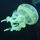# Does anyone know what this number is called?

Asked by doyendroll (212) 2 weeks ago

I was thinking of a number and I decided to multiply it by itself. Then I added 1, as can often happen. Next I added twice the original number to the tally. Feeling somewhat exhilarated I exponentiated the lot to the power 0.5 after which I subtracted the original number. Finally I subtracted 2 then found the square root of that result. All that was left is a letter. I would like to give it a name. Any suggestions?

Observing members: 0Composing members: 0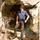X

kritiper (18904)“Great Answer” (1) Flag as…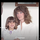Y

Dutchess_lll (8466)“Great Answer” (1) Flag as…Dutchess_lll (8466)“Great Answer” (1) Flag as…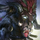How about i (aka “the imaginary unit” or “unit imaginary number”)?

√(((((n^2 + 1) + 2n)^0.5) - n) - 2) = i

1×1 = 1
1 + 1 = 2
2 + 2 = 4
4 ^ .05 = 2
2 – 1 = 1
1 – 2 = -1
√-1 = i

Irukandji (3211)“Great Answer” (2) Flag as…Aye

doyendroll (212)“Great Answer” (0) Flag as…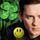I get it. Very clever! Thanks!

LuckyGuy (38056)“Great Answer” (2) Flag as…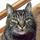This is FANTASTIC!!!!
Reminds me of e to the X power.

gondwanalon (18390)“Great Answer” (1) Flag as…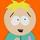WTF

filmfann (47290)“Great Answer” (1) Flag as…Just a quick note. If you remember your high school algebra, you should be able to tell that the answer is the same for any value of n.

For starters, ( n**2 + 1 + 2n)**0.5 = √(n+1)**2 = n+1. I will let you work out the rest.

LostInParadise (27386)“Great Answer” (2) Flag as…Here is a simple process that mathematicians have not figured out. Start with any number. If it is odd, multiply by 3 and add 1. If it is even, divide by 2/ Keep repeating this procedure. So far, it has always reached 1, at which point you keep cycling through the numbers 4, 2 and 1. Nobody can explain why it always ends this way.

LostInParadise (27386)“Great Answer” (1) Flag as…Response moderated